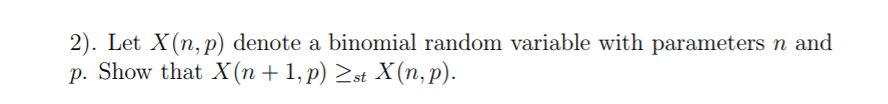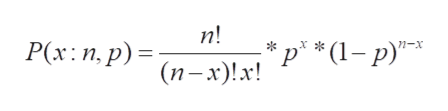2). Let X(n, p) denote a binomial random variable with parameters n andp. Show that X (n 1,p) st X (n, p)

Question

See attachment.help_outlineImage Transcriptionclose2). Let X(n, p) denote a binomial random variable with parameters n and p. Show that X (n 1,p) st X (n, p) fullscreen
Step 1

Given X(n,p) is a binomial random variable with parameters n,p.
n is total number of trails
p is probability of success.
The probability distribution function of binomial distribution for k number of success with parameters n,p is shown belowhelp_outlineImage Transcriptionclosen! Р(х: п,р) - (п-х)!! Р * (1— р)" fullscreen
Step 2

Any binomial random variable with low p and low n values distribution is left tail, for large p and small n values, distribution is right tail and for large n and for p = 0.5 the distr...

Want to see the full answer?

See Solution

Want to see this answer and more?

Our solutions are written by experts, many with advanced degrees, and available 24/7

See Solution
Tagged in

Basic Probability